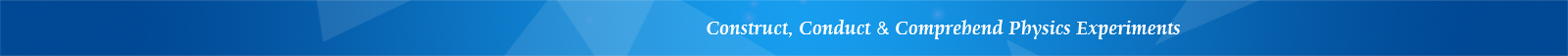## LETI-20 Thermal Conductivity Measurement Apparatus

Features

• High performance temperature controller and sensor

• Simple structure, stable and reliable

• Affordable

Introduction

Thermal conductivity coefficient is a physical quantity representing the heat conduction characteristics of a material. Material structure change and impurities have a significant impact on thermal conductivity, so the thermal conductivity of a material often needs to be experimentally measured. Experimental methods for measuring thermal conductivity are divided into two categories: steady-state method and dynamic method. A steady-state method uses a heating source to heat the specimen, and the temperature at different areas inside the specimen depends on the heating speed and the heat conduction speed of the material. When the experimental conditions and parameters are properly controlled to make the heating and heat transfer process reach a balanced state, a stable temperature distribution inside the material will be formed. Based on the temperature distribution, the thermal conductivity coefficient of the material can be calculated.

This experiment measures the thermal conductivity coefficient of a poor conductor (rubber or bakelite) by using the steady-state method. Students can learn how to calculate the transfer rate and the cooling rate. The apparatus uses an adaptive heating plate with microcomputer temperature control, yielding a reading resolution of 0.1 °C. The instrument has been widely used in thermal physics experiments at universities.

Using this apparatus, the following experiments can be performed:

1. Measure thermal conductivity of poor conductor (rubber and bakelite specimens included)

2. Learn how to calculate heat conduction rate using cooling rate

3. Learn how to use an integrated temperature sensor